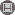دوره 10، شماره 2 - ( 7-1394 )                   جلد 10 شماره 2 صفحات 1-10 | برگشت به فهرست نسخه ها

BibTeX | RIS | EndNote | Medlars | ProCite | Reference Manager | RefWorks
Send citation to:Hashemi Borzabadi A, Heidari M. A Successive Numerical Scheme for Some Classes of Volterra-Fredholm Integral Equations. IJMSI. 2015; 10 (2) :1-10
URL: http://ijmsi.ir/article-1-793-fa.html
A Successive Numerical Scheme for Some Classes of Volterra-Fredholm Integral Equations. مجله علوم ریاضی و انفورماتیک. 1394; 10 (2) :1-10

چکیده:

In this paper, a reliable iterative approach, for solving a wide range of linear and nonlinear Volterra-Fredholm integral equations is established. First the approach considers a discretized form of the integral terms where considering some conditions on the kernel of the integral equation it is proved that solution of the discretized form converges to the exact solution of the problem. Then the solution of the discretized form is approximated by an iterative scheme. Comparison of the approximate solution with exact solution shows that the used approach is easy and practical for some classes of linear and nonlinear Volterra-Fredholm integral equations.

نوع مطالعه: پژوهشي | موضوع مقاله: تخصصي

ارسال نظر درباره این مقاله : نام کاربری یا پست الکترونیک شما:کلیه حقوق این وب سایت متعلق به نشریه علوم ریاضی و انفورماتیک می باشد.

طراحی و برنامه نویسی : یکتاوب افزار شرق